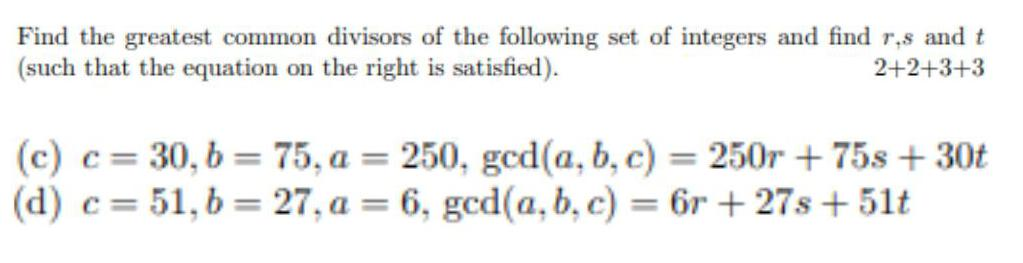Question:

# Find the greatest common divisors of the following set of

Last updated: 7/30/2022Find the greatest common divisors of the following set of integers and find r.s and t (such that the equation on the right is satisfied). 2+2+3+3 (c) c = 30,b= 75, a = 250, gcd(a, b, c) = 250r +75s + 30t (d) c= 51, b = 27, a = 6, gcd(a, b, c) = 6r+27s+ 51t